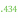# LOG10E

 Block Group: Constants Icon:The LOG10E block is a Number block. When the LOG10E block is created, the LOG10E block holds the mathematical constant for the base-10 logarithm of the constant e.

For information on using dataflow blocks, see Dataflow.

## Input/Output Property

The following property of the LOG10E block can take input and give output.

• value (number)

value sets and returns the number held by this LOG10E block. When a LOG10E block is created, the value property holds the mathematical constant for the base-10 logarithm of the constant e.

## Example

The following image demonstrates an example of the LOG10E block.